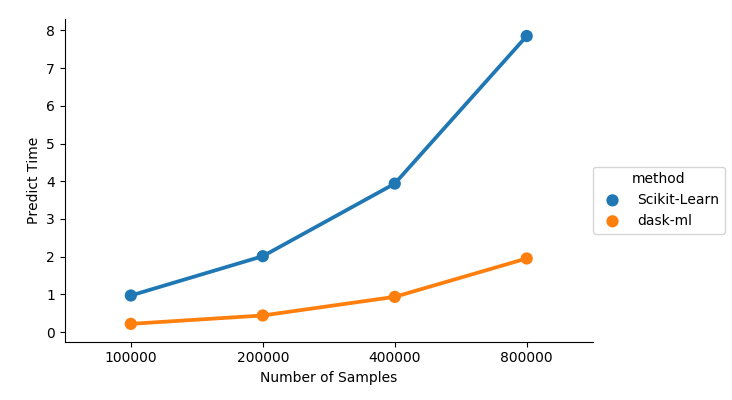# Parallelizing Predicion¶

This example demonstrates `dask_ml.wrappers.ParallelPostFit`. A `sklearn.svm.SVC` is fit on a small dataset that easily fits in memory.

After training, we predict for successively larger datasets. We compare

• The serial prediction time using the regular SVC.predict method
• The parallel prediction time using `dask_ml.warppers.ParallelPostFit.predict()`

We see that the parallel version is faster, especially for larger datasets. Additionally, the parallel version from ParallelPostFit scales out to larger than memory datasets.

While only predict is demonstrated here, wrappers.ParallelPostFit is equally useful for predict_proba and transform.```from timeit import default_timer as tic

import pandas as pd
import seaborn as sns
import sklearn.datasets
from sklearn.svm import SVC

X, y = sklearn.datasets.make_classification(n_samples=1000)
clf = ParallelPostFit(SVC(gamma='scale'))
clf.fit(X, y)

Ns = [100_000, 200_000, 400_000, 800_000]
timings = []

for n in Ns:
random_state=n,
chunks=n // 20)
t1 = tic()
# Serial scikit-learn version
clf.estimator.predict(X)
timings.append(('Scikit-Learn', n, tic() - t1))

t1 = tic()
# Parallelized scikit-learn version
clf.predict(X).compute()